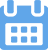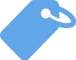# The Meaning of PEMDAS: What It Is and How To Use It

•Kevin
•March 27, 2022
•Do you know how to use PEMDAS in mathematical calculations? Keep reading to learn all about the meaning of PEMDAS and how to use it.### Your writing, at its best

Compose bold, clear, mistake-free, writing with Grammarly's AI-powered writing assistant

A lot of us learned about PEMDAS in grade school math, but do you remember what PEMDAS stands for and how it is used to calculate complex equations? Keep reading to learn what PEMDAS stands for, how to remember PEMDAS, and examples of PEMDAS.

## What Does the Word PEMDAS Mean?

According to Math is Fun, PEMDAS is a mnemonic device that is used to remember the mathematical order of operations. PEMDAS stands for Parentheses, Exponent, Multiply, Divide, Add, and Subtract. The abbreviation for this set of rules is used to avoid confusion in complex equations and ensure that math students get the correct final answer.

Different countries like Canada, India, and the UK call PEMDAS “BEDMAS” or “BODMAS” where the B stands for brackets instead of parentheses or “GEMDAS” where the G stands for grouping symbols. There are many acronyms and names for PEMDAS, but the fixed sequence stays the same.

Math students learn PEMDAS in grade school, but this mnemonic is used for even the most complex math. The order of operations is used in fields like physics and programming languages to preserve key nuances and ensure people do not get different answers.

Often, people remember this mnemonic with the phrase “Please Excuse My Dear Aunt Sally.” However, there are many other acronyms that people use to remember the order of the letters in the PEMDAS rule. The Free Dictionary lists some of these below:

• Pink Elephants Marching Down a Street
• Please Excuse My Dumb Ass Students
• Purple Eggplants Make Delicious Afternoon Snacks
• Please Excuse My Dumb Ass Sister
• People Expect More Drugs and Sex
• People Eat More Donuts After School
• Please End My Day At School
• Pink Elephants Make Dandy Apple Sauce
• Purple Elephants Marching Down A Street
• Peanuts Ended My Donut Addiction Saturday
• Picking Eminem Made Dre a Star
• Purple Elephants May Destroy a School
• Please Excuse My Dope Ass Swag
• Pancake Explosion Many Deaths Are Suspected
• Please End My Depression and Suffering

### Parentheses

If parts of a math problem are in parentheses, curly braces, or square brackets, you will calculate those first. For example, things in parentheses could include addition, subtraction, multiplication, and division.

For example, if your math problem is:

5 + (6 x 2) = 5 + 12 = 17

You would have to do the “6 x 2” in the parentheses first. Otherwise, you would get the below (wrong) answer by not following the order of operations:

5 + (6 x 2) = 11 x 2 = 22

### Exponents

Suppose you see parts of a math problem that have powers, roots, or other forms of exponentiation. In that case, you will perform the next series of calculations after parentheses but before multiplying, dividing, adding, or subtracting.

For example, if your math problem is:

3 + 42 = 3 + 16 = 19

You must calculate 4 to the 2nd power first before adding it to the number 3. Otherwise, by not following the order of operations, you end up with the incorrect answer below:

3 + 42 = 72 = 49

### Multiply and Divide

Third in the order of operations is multiplication and division. In a math problem, you will complete all of the multiplication and division at once by performing the calculations from left to right.

For example, if your math problem is:

10 – 2 x 4 = 10 – 8 = 2

You must first calculate 2 x 4. Then, you will subtract that number from the number 10. Otherwise, if you ignore the order of operations and simply calculate from left to right, you end up with the incorrect calculation below:

10 – 2 x 4 = 8 x 4 = 32

Finally, we have addition and subtraction. Like multiplication and division, you should complete addition and subtraction from left to right, regardless if addition or subtraction comes first.

For example, if your math problem is:

9 – 4 + 3 = 5 + 3 = 8

Even though the subtraction is first and the addition is second, you will still calculate them from left to right. Otherwise, if you add and then subtract, you end up with this incorrect answer:

9 – 4 + 3 = 9 – 7 = 2

## What Are Translations of PEMDAS?

Acronyms can be tricky to translate to other languages because words in different languages might start with different letters. However, Nice Translator lists many translations for the term order of operations, which can be used in place of PEMDAS.

• Hebrew: סדר פעולות
• Urdu: آپریشن کا حکم
• Portuguese (Brazil): ordem de operações
• Hungarian: műveletek sorrendje
• Malay: perintah operasi
• Italian: ordine delle operazioni
• Estonian: Toimingute järjekord
• Dutch: volgorde van operaties
• Turkish: operasyonların sırası
• Greek: σειρά πράξεων
• Icelandic: röð af rekstri
• Finnish: Toimintajärjestys
• Romanian: ordinea operațiunilor
• Russian: Порядок операций
• Hindi: कार्रवाई के आदेश
• Korean: 운영 명령
• Spanish: Orden de operaciones
• Thai: คำสั่งของการดำเนินงาน
• Swahili: utaratibu wa uendeshaji
• Lithuanian: Operacijų tvarka
• Bulgarian: ред на операциите
• Croatian: red operacija
• Slovenian: Organ
• Vietnamese: trật tự của hoạt động
• Japanese: 営業順
• Chinese (Taiwan): 操作順序
• Polish: kolejność operacji
• Amharic: የክዋኔዎች ቅደም ተከተል
• Norwegian: Operasjonsordre
• Indonesian: Urutan operasi
• Telugu: ఆపరేషన్స్ ఆర్డర్
• Tamil: நடவடிக்கைகளின் ஆணை
• French: ordre des opérations
• Basque: Eragiketen ordena
• Latvian: Darbības kārtība
• Marathi: ऑपरेशन ऑर्डर
• Swedish: OBSORT
• Chinese (PRC): 操作顺序
• Catalan: Ordre d’operacions
• Serbian: редослед пословања
• German: Reihenfolge der Operationen
• Arabic: ترتيب العمليات
• Danish: ordre af operationer
• Bengali: অপারেশন অর্ডার
• Malayalam: പ്രവർത്തന ക്രമം
• Portuguese (Portugal): ordem de operações
• Ukrainian: Порядок операцій
• Gujarati: ઓપરેશન્સનો ક્રમ

## Conclusion

PEMDAS stands for Parentheses, Exponent, Multiply & Divide, Add & Subtract. This is known as the order of operations in math.